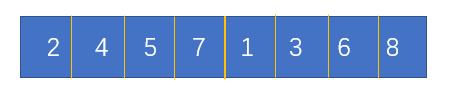# 求逆序对的方法【逆序对】【树状数组】【归并排序】

题面可见洛谷P1908逆序对

逆序对，最朴素的做法就是$O(N^2)$的了，枚举每个数对，逆序则sum++。

不过主流做法是归并排序，也可以用树状数组（线段树）来做。在这个图中，前4个和后4个都是有序的。所以逆序对只会在前面的数与后面比它小的数中出现。我们把这个数组看作两个栈，s1和s2。

第一个出栈的是s2的‘1’，此时在s1的一定全部比它大。所以将逆序对的数目加上s1.size()。（实际应用中加的是数组的长度）

所以在后面元素出栈时，给逆序对个数带来的贡献分别是：1：4   3：3   6：1   8：0。

之后这个序列就是有序的了，它的内部不再有逆序对。

### Code：

#include<iostream>
using namespace std;
int a;
int b;
unsigned long long int sum=0;
int num;
void merging(int l1,int r1,int l2,int r2)
{
int i=l1;
int j=l2;
int k=l1;
while(i<=r1&&j<=r2)
{
if(a[i]<=a[j])//求逆序对
b[k++]=a[i++];
else
{
b[k++]=a[j++];
sum++;
sum+=r1;
sum-=i;
}
}
while(i<=r1)
b[k++]=a[i++];
while(j<=r2)
b[k++]=a[j++];
for(int i=l1;i<=r2;i++)
a[i]=b[i];
}
void merged(int l,int r)
{
if(l==r)
return;
int mid;
mid=(l+r)/2;
merged(l,mid);
merged(mid+1,r);
merging(l,mid,mid+1,r);
}
int main(void)
{
cin>>num;
for(int i=1;i<=num;i++)
cin>>a[i];
merged(1,num);
cout<<sum;
}


树状数组的做法看上去要直观一些，比归并排序稍微好理解一点，但是需要$Nlog N$的预处理。这样一来常数就基本是2了。

首先，我们要对被处理数组离散化，重复的数字映射为同一个象。然后建立长度为n的树状数组（需满足操作单点增加和区间求和），初始化为0。

然后，从前到后扫描被处理数组。设当前为第k个数，那么a[k]的象为$f(a[k])$，当扫描到k时，查询在这个数前面（也就是已经被处理的1到k-1）出现过多少个比a[k]大的数，等价于求有多少个象比$f(a[k])$大的原象，就是这个数与它前面的数所产生的所有逆序对了。完成这个工作后将树状数组上的$f(a[k])$加一。

比如说一串数字（已经离散化）5 2 4 3 1

c    c    c    c    c    sum

### Code：

#include<cstdio>
#include<cstring>
#include<algorithm>
#include<iostream>
using std::pair;
int c;
bool flag;
pair<int,int> a;
bool cmp(pair<int,int> x,pair<int,int> y)
{
return x.second<y.second;
}
int n;
int lowbit(int x)
{
return x&(-x);
}
{
while(t<=n)
{
c[t]+=x;
t+=lowbit(t);
}
return;
}
{
int sum=0;
while(x>0)
{
sum+=c[x];
x-=lowbit(x);
}
return sum;
}
int main()
{
memset(flag,0,sizeof(flag));
scanf("%d",&n);
for(int i=1;i<=n;i++)
{
scanf("%d",&a[i].first);
a[i].second=i;
}
std::sort(a+1,a+1+n);
a.first=0;
for(int i=1;i<=n;i++)
if(a[i].first==a[i-1].first)
flag[i]=true;
for(int i=1;i<=n;i++)
if(flag[i])
a[i].first=a[i-1].first;
else
a[i].first=i;
std::sort(a+1,a+1+n,cmp);
memset(c,0,sizeof(c));
int sum=0;
for(int i=1;i<=n;i++)
{
}
printf("%d\n",sum);
return 0;
}


### 说点什么Subscribe

/* */